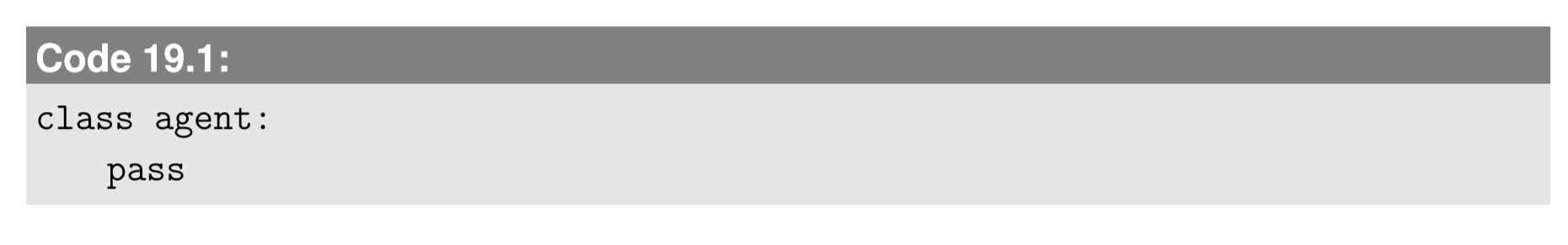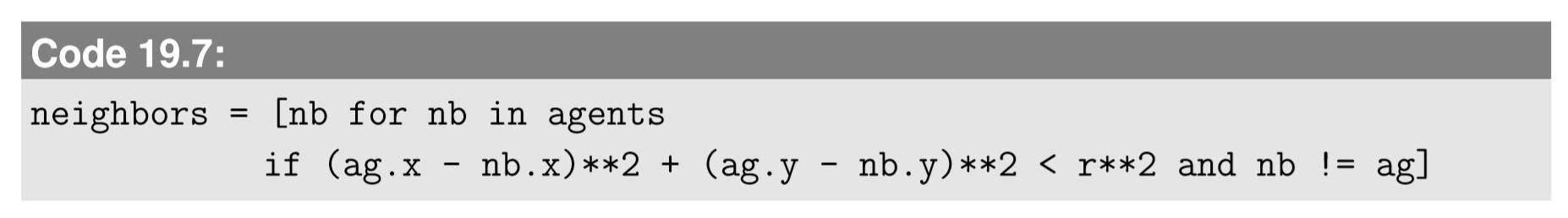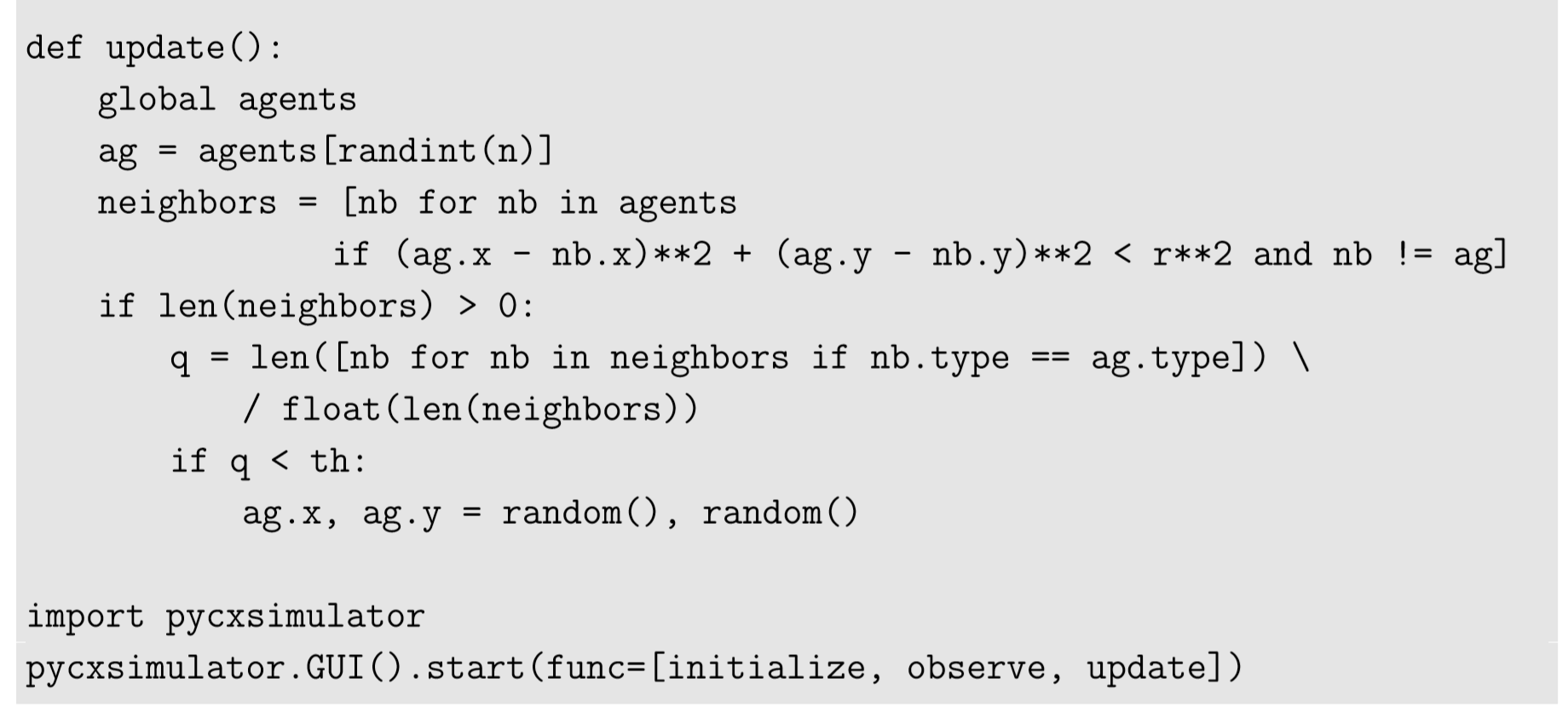Skip to main content
$$\newcommand{\id}{\mathrm{id}}$$ $$\newcommand{\Span}{\mathrm{span}}$$ $$\newcommand{\kernel}{\mathrm{null}\,}$$ $$\newcommand{\range}{\mathrm{range}\,}$$ $$\newcommand{\RealPart}{\mathrm{Re}}$$ $$\newcommand{\ImaginaryPart}{\mathrm{Im}}$$ $$\newcommand{\Argument}{\mathrm{Arg}}$$ $$\newcommand{\norm}{\| #1 \|}$$ $$\newcommand{\inner}{\langle #1, #2 \rangle}$$ $$\newcommand{\Span}{\mathrm{span}}$$

# 9.2: Building an Agent-Based Model

$$\newcommand{\vecs}{\overset { \rightharpoonup} {\mathbf{#1}} }$$

$$\newcommand{\vecd}{\overset{-\!-\!\rightharpoonup}{\vphantom{a}\smash {#1}}}$$

Let’s get started with agent-based modeling. In fact, there are many great tutorials already out there about how to build an ABM, especially those by Charles Macal and Michael North, renowned agent-based modelers at Argonne National Laboratory . Macal and North suggest considering the following aspects when you design an agent-based model:

1. Speciﬁc problem to be solved by the ABM
2. Design of agents and their static/dynamic attributes
3. Design of an environment and the way agents interact with it
4. Design of agents’ behaviors
5. Design of agents’ mutual interactions
6. Availability of data
7. Method of model validation

Among those points, 1, 6, and 7 are about fundamental scientiﬁc methodologies. It is important to keep in mind that just building an arbitrary ABM and obtaining results by simulation wouldn’t produce any scientiﬁcally meaningful conclusion. In order for an ABM to be scientiﬁcally meaningful, it has to be built and used in either of the following two complementary approaches:

1. Build an ABM using model assumptions that are derived from empirically observed phenomena, and then produce previously unknown collective behaviors by simulation.
2. Build an ABM using hypothetical model assumptions, and then reproduce empirically observed collective phenomena by simulation.

The former is to use ABMs to make predictions using validated theories of agent behaviors, while the latter is to explore and develop new explanations of empirically observed phenomena. These two approaches are different in terms of the scales of the known and the unknown (A uses micro-known to produce macro-unknown, while B uses micro-unknown to reproduce macro-known), but the important thing is that one of those scales should be grounded on well-established empirical knowledge. Otherwise, the simulation results would have no implications for the real-world system being modeled. Of course, a free exploration of various collective dynamics by testing hypothetical agent behaviors to generate hypothetical outcomes is quite fun and educational, with lots of intellectual beneﬁts of its own. My point is that we shouldn’t misinterpret outcomes obtained from such exploratory ABMs as a validated prediction of reality.

In the meantime, items 2, 3, 4, and 5 in Macal and North’s list above are more focused on the technical aspects of modeling. They can be translated into the following design tasks in actual coding using a programming language like Python:

Design tasks you need to do when you implement an ABM

1. Design the data structure to store the attributes of the agents.
2. Design the data structure to store the states of the environment.
3. Describe the rules for how the environment behaves on its own.
4. Describe the rules for how agents interact with the environment.
5. Describe the rules for how agents behave on their own.
6. Describe the rules for how agents interact with each other.

## Representation of agents in Python

It is often convenient and customary to deﬁne both agents’ attributes and behaviors using a class in object-oriented programming languages, but in this textbook, we won’t cover object-oriented programming in much detail. Instead, we will use Python’s dynamic class as a pure data structure to store agents’ attributes in a concise manner. For example, we can deﬁne an empty agent class as follows:The class command deﬁnes a new class under which you can deﬁne various attributes (variables, properties) and methods (functions, actions). In conventional object-oriented programming, you need to give more speciﬁc deﬁnitions of attributes and methods available under this class. But in this textbook, we will be a bit lazy and exploit the dynamic, ﬂexible nature of Python’s classes. Therefore, we just threw pass into the class deﬁnition above. pass is a dummy keyword that doesn’t do anything, but we still need something there just for syntactic reasons.

Anyway, once this agent class is deﬁned, you can create a new empty agent object as follows:Then you can dynamically add various attributes to this agent object a:This ﬂexibility is very similar to the ﬂexibility of Python’s dictionary. You don’t have to predeﬁne attributes of a Python object. As you assign a value to an attribute (written as “object’s name”.“attribute”), Python automatically generates a new attribute if it hasn’t been deﬁned before. If you want to know what kinds of attributes are available under an object, you can use the dir command:The ﬁrst two attributes are Python’s default attributes that are available for any objects (you can ignore them for now). Aside from those, we see there are four attributes deﬁned for this object a.

n the rest of this chapter, we will use this class-based agent representation. The technical architecture of simulator codes is still the same as before, made of three components: initialization, visualization, and updating functions. Let’s work on some examples to see how you can build an ABM in Python.

## Schelling’s Segregation Model

There is a perfect model for our ﬁrst ABM exercise. It is called Schelling’s segregation model, widely known as the very ﬁrst ABM proposed in the early 1970s by Thomas Schelling, the 2005 Nobel Laureate in Economics . Schelling created this model in order to provide an explanation for why people with different ethnic backgrounds tend to segregate geographically. Therefore, this model was developed in approach B discussed above, reproducing the macro-known by using hypothetical micro-unknowns. The model assumptions Schelling used were the following:

• Two different types of agents are distributed in a ﬁnite 2-D space.
• In each iteration, a randomly chosen agent looks around its neighborhood, and if the fraction of agents of the same type among its neighbors is below a threshold, it jumps to another location randomly chosen in the space.

As you can see, the rule of this model is extremely simple. The main question Schelling addressed with this model was how high the threshold had to be in order for segregation to occur. It may sound reasonable to assume that segregation would require highly homophilic agents, so the critical threshold might be relatively high, say 80% or so. But what Schelling actually showed was that the critical threshold can be much lower than one would expect. This means that segregation can occur even if people aren’t so homophilic. In the meantime, quite contrary to our intuition, a high level of homophily can actually result in a mixed state of the society because agents keep moving without reaching a stationary state. We can observe these emergent behaviors in simulations.

Back in the early 1970s, Schelling simulated his model on graph paper using pennies and nickels as two types of agents (this was still a perfectly computational simulation!). But here, we can loosen the spatial constraints and simulate this model in a continuous space. Let’s design the simulation model step by step, going through the design tasks listed above.

1. Design the data structure to store the attributes of the agents. In this model, each agent has a type attribute as well as a position in the 2-D space. The two types can be represented by 0 and 1, and the spatial position can be anywhere within a unit square. Therefore we can generate each agent as follows:To generate a population of agents, we can write something like:2. Design the data structure to store the states of the environment, 3. Describe the rules for how the environment behaves on its own, & 4. Describe the rules for how agents interact with the environment. Schelling’s model doesn’t have a separate environment that interacts with agents, so we can skip these design tasks.

5. Describe the rules for how agents behave on their own. We assume that agents don’t do anything by themselves, because their actions (movements) are triggered only by interactions with other agents. So we can ignore this design task too.

6. Describe the rules for how agents interact with each other. Finally, there is something we need to implement. The model assumption says each agent checks who are in its neighborhood, and if the fraction of the other agents of the same type is less than a threshold, it jumps to another randomly selected location. This requires neighbor detection, which was easy in CA and networks because the neighborhood relationships were explicitly modeled in those modeling frameworks. But in ABM, neighborhood relationships may be implicit, which is the case for our model. Therefore we need to implement a code that allows each agent to ﬁnd who is nearby.

There are several computationally efﬁcient algorithms available for neighbor detection, but here we use the simplest possible method: Exhaustive search. You literally check all the agents, one by one, to see if they are close enough to the focal agent. This is not computationally efﬁcient (its computational amount increases quadratically as the number of agents increases), but is very straightforward and extremely easy to implement. You can write such exhaustive neighbor detection using Python’s list comprehension, e.g.:Here ag is the focal agent whose neighbors are searched for. The if part in the list comprehension measures the distance squared between ag and nb, and if it is less than r squared (r is the neighborhood radius, which must be deﬁned earlier in the code) nb is included in the result. Also note that an additional condition nb != ag is given in the if part. This is because if nb == ag, the distance is always 0 so ag itself would be mistakenly included as a neighbor of ag.

Once we obtain neighbors for ag, we can calculate the fraction of the other agents whose type is the same as ag’s, and if it is less than a given threshold, ag’s position is randomly reset. Below is the completed simulator code, with the visualization function also implemented using the simple plot function:When you run this code, you should set the step size to 50 under the “Settings” tab to speed up the simulations.

Figure 19.2.1 shows the result with the neighborhood radius r=0.1 and the threshold for moving th = 0.5. It is clearly observed that the agents self-organize from an initially random distribution to a patchy pattern where the two types are clearly segregated from each other.Figure $$\PageIndex{1}$$: Visual output of Code 19.8. Time ﬂows from left to right.

Exercise $$\PageIndex{1}$$

Conduct simulations of Schelling’s segregation model with th (threshold for moving), r (neighborhood radius), and/or n (population size = density) varied systematically. Determine the condition in which segregation occurs. Is the transition gradual or sharp?

Exercise $$\PageIndex{2}$$

Develop a metric that characterizes the level of segregation from the positions of the two types of agents. Then plot how this metric changes as parameter values are varied.

Here are some other well-known models that show quite unique emergent patterns or dynamic behaviors. They can be implemented as an ABM by modifying the code for Schelling’s segregation model. Have fun!

Exercise $$\PageIndex{3}$$: Diffusion-limited aggregation

Diffusion-limited aggregation (DLA) is a growth process of clusters of aggregated particles driven by their random diffusion. There are two types of particles, like in Schelling’s segregation model, but only one of them can move freely. The movable particles diffuse in a 2-D space by random walk, while the immovable particles do nothing; they just remain where they are. If a movable particle “collides” with an immovable particle (i.e., if they come close enough to each other), the movable particle becomes immovable and stays there forever. This is the only rule for the agents’ interaction.

Implement the simulator code of the DLA model. Conduct a simulation with all particles initially movable, except for one immovable “seed” particle placed at the center of the space, and observe what kind of spatial pattern emerges. Also carry out the simulations with multiple immovable seeds randomly positioned in the space, and observe how multiple clusters interact with each other at macroscopic scales.

For your information, a completed Python simulator code of the DLA model is available from http://sourceforge.net/projects/pycx/files/, but you should try implementing your own simulator code ﬁrst.

Exercise $$\PageIndex{4}$$: Boids

This is a fairly advanced, challenging exercise about the collective behavior of animal groups, such as bird ﬂocks, ﬁsh schools, and insect swarms, which is a popular subject of research that has been extensively modeled and studied with ABMs. One of the earliest computational models of such collective behaviors was proposed by computer scientist Craig Reynolds in the late 1980s. Reynolds came up with a set of simple behavioral rules for agents moving in a continuous space that can reproduce an amazingly natural-looking ﬂock behavior of birds. His model was called bird-oids, or “Boids” for short. Algorithms used in Boids has been utilized extensively in the computer graphics industry to automatically generate animations of natural movements of animals in ﬂocks (e.g., bats in the Batman movies). Boids’ dynamics are generated by the following three essential behavioral rules (Fig. 19.2.1):

• Cohesion Agents tend to steer toward the center of mass of local neighbors.
• Alignment Agents tend to steer to align their directions with the average velocity of local neighbors.
• Separation Agents try to avoid collisions with local neighbors.

Design an ABM of collective behavior with these three rules, and implement its simulator code. Conduct simulations by systematically varying relative strengths of the three rules above, and see how the collective behavior changes.

You can also simulate the collective behavior of a population in which multiple types of agents are mixed together. It is known that interactions among kinetically distinct types of swarming agents can produce various nontrivial dynamic patterns .Figure $$\PageIndex{2}$$ Three essential behavioral rules of Boids. (a) Cohesion. (b) Alignment. (c) Separation.